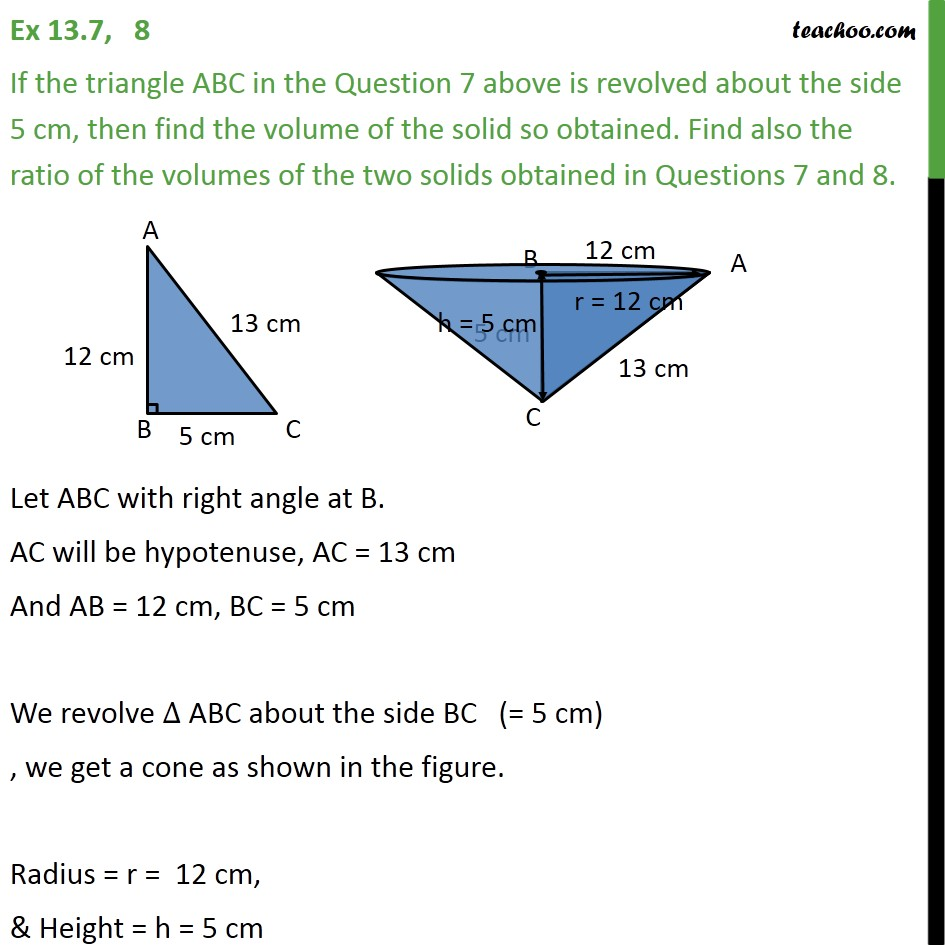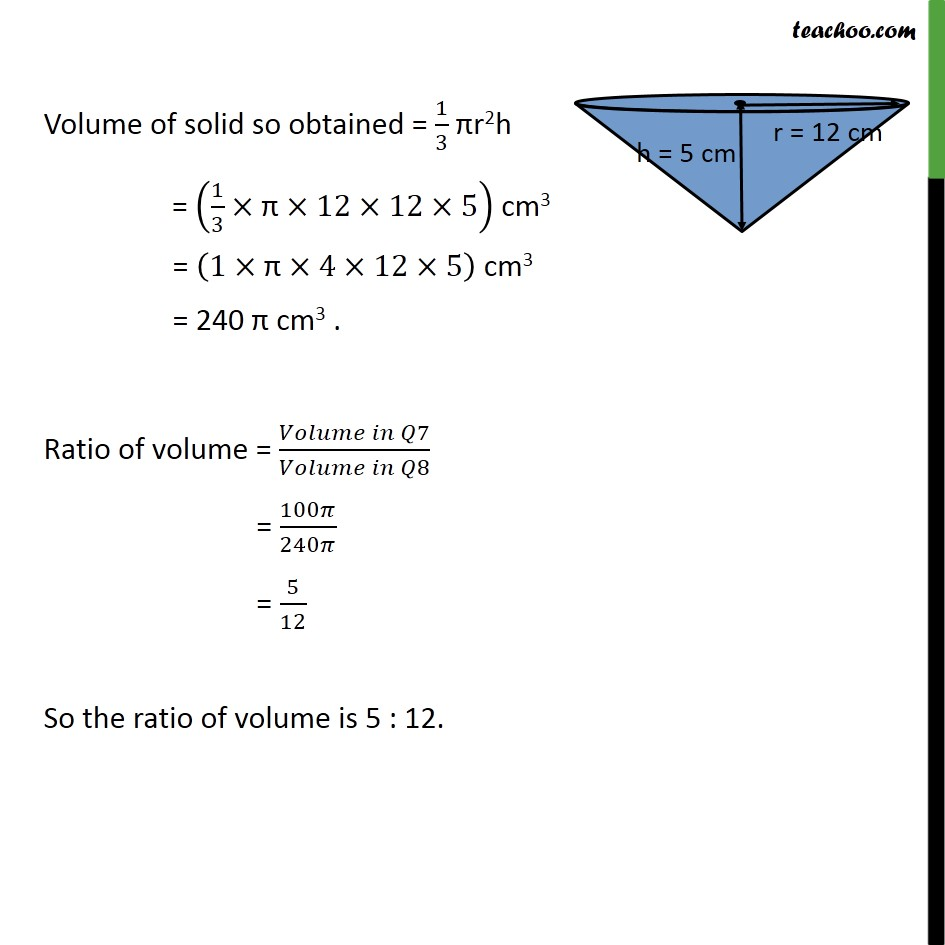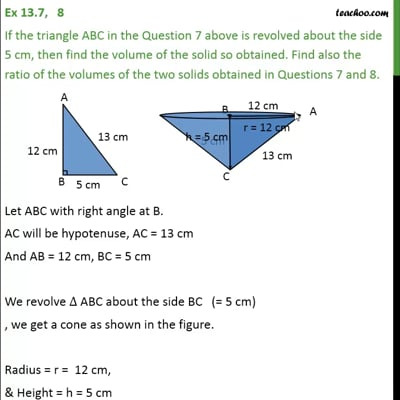Ex 13.7

Chapter 13 Class 9 Surface Areas and Volumes
Serial order wiseThis video is only available for Teachoo black users

Maths Crash Course - Live lectures + all videos + Real time Doubt solving!

### Transcript

Ex 13.7, 8 If the triangle ABC in the Question 7 above is revolved about the side 5 cm, then find the volume of the solid so obtained. Find also the ratio of the volumes of the two solids obtained in Questions 7 and 8. Let ABC with right angle at B. AC will be hypotenuse, AC = 13 cm And AB = 12 cm, BC = 5 cm We revolve ∆ ABC about the side BC (= 5 cm) , we get a cone as shown in the figure. Radius = r = 12 cm, & Height = h = 5 cm Volume of solid so obtained = 1/3 πr2h = (1/3×"π" ×12×12×5) cm3 = (1×"π" ×4×12×5) cm3 = 240 π cm3 . Ratio of volume = (𝑉𝑜𝑙𝑢𝑚𝑒 𝑖𝑛 𝑄7)/(𝑉𝑜𝑙𝑢𝑚𝑒 𝑖𝑛 𝑄8) = 100𝜋/240𝜋 = 5/12 So the ratio of volume is 5 : 12.